# What to do with errors during modal calculation?

The following errors could appear during a modal calculation and will be discussed using a simple example: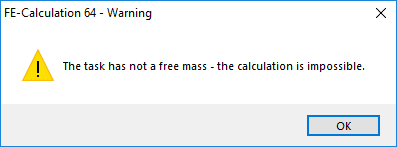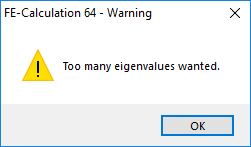Suppose a simply supported beam, for which a message appears at the start of the modal analysis: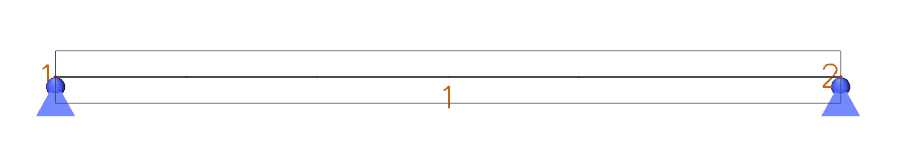By default, there is only one finite mesh element created per beam. This means that the mass of the beam will be completely divided to the two end nodes, which are both rigidly supported for displacements. Therefore, the mass cannot move, which results in zero degrees of freedom and the following error message: “The task has not a free mass – the calculation is impossible”.The solution is to increase the average number of mesh elements for 1D elements via Setup > Mesh: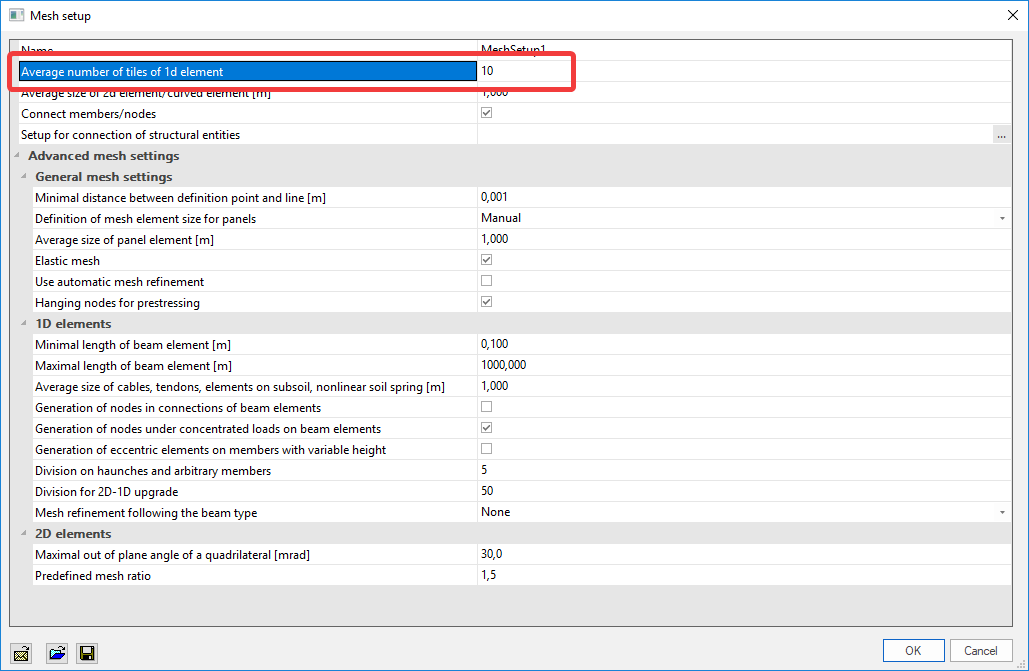If this parameter is increased to 10, this results in a higher amount of degrees of freedom because there are more nodes available to distribute the mass:Another error message that can appear is “Too many eigenvalues wanted”:This means that the requested number of eigenvalues cannot be found. First of all, you can increase the number of mesh elements for 1D-elements if necessary. This value should be high enough for the mass to be distributed correctly, as mentioned above.

If this value is sufficient, but the error message still appears, one of the following options can help:

• Reduce the number of requested eigenvalues
• Reduce de requested solver precision ratio
• Use an alternative method, e.g. subspace iteration

These options can be changed in the solver settings via Setup > Solver: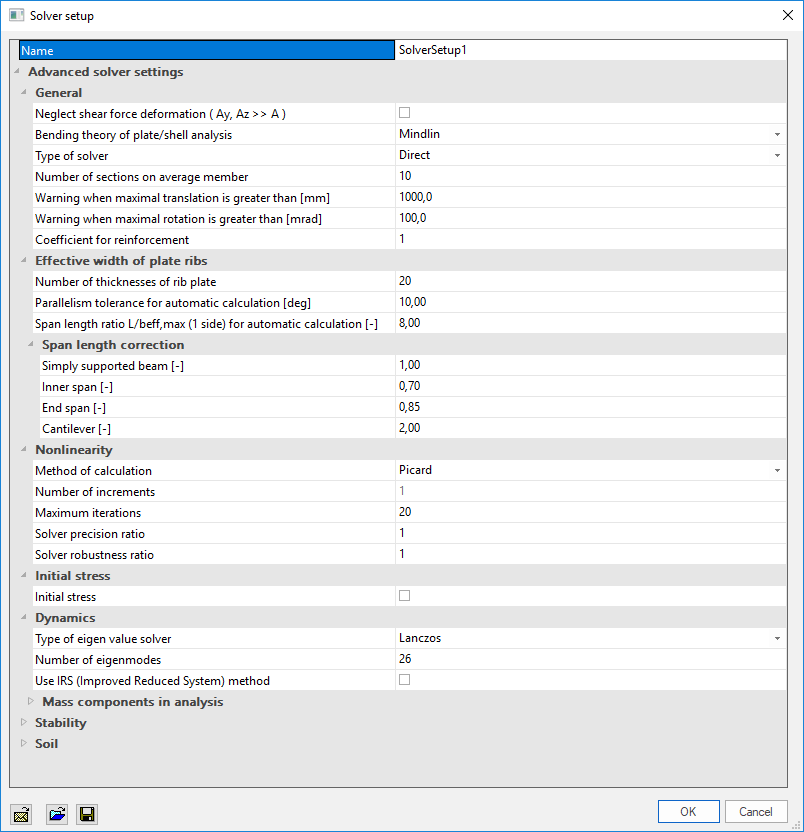Remark: In previous version of SCIA Engineer, these error messages could be different. E.g.: “Number of the nonzero numbers in the mass vector should be at least twice as big as it is” or “Insufficient number of resulting eigenvalues”